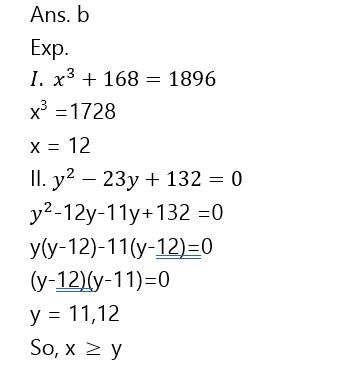# SBI PO Mains Quantitative Aptitude Quiz- 5

## SBI PO Mains Quantitative Aptitude Quiz

Quantitative aptitude measures a candidate’s numerical proficiency and problem-solving abilities. It is the most important section of almost all competitive exams. Candidates are often stymied by the complexity of Quantitative Aptitude Questions but if they practice more and more questions it will become quite easy. So, here we are providing you with the SBI PO Mains Quantitative Aptitude Quiz to enhance your preparation for your upcoming examination. Questions given in this SBI PO Mains Quantitative Aptitude Quiz are based on the most recent and the latest exam pattern. A detailed explanation for each question will be given in this SBI PO Mains Quantitative Aptitude Quiz. This SBI PO Mains Quantitative Aptitude Quiz is entirely free of charge. This SBI PO Mains Quantitative Aptitude Quiz will assist aspirants in achieving a good score in their upcoming examinations.

Directions (1-5): Following pie chart 1 and pie chart 2 shows the total number of students appeared in Bank PO exam in six different states in 2018 and 2019 respectively. Study the pie charts carefully and answer the following questions.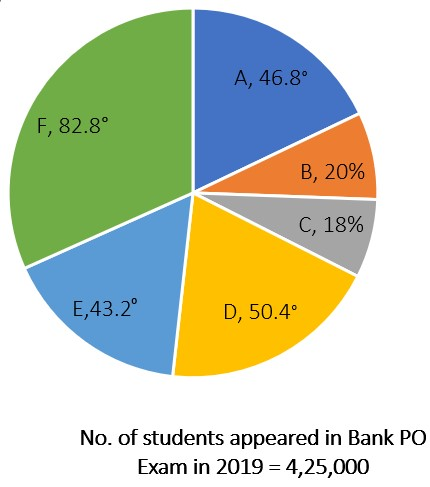1. Number of students appeared in Bank PO exam from state A in 2019 are approximately what percent of number of students appeared in Bank PO exam from state B in 2018?

(a)82%

(b)79%

(c)72%

(d)87%

(e)76%1. What is the difference between number of students appeared in Bank PO exam from state E in 2019 and number of students appeared in Bank PO exam from state B in 2018?

(a) 15000

(b) 17000

(c) 17500

(d) 16500

(e) 16000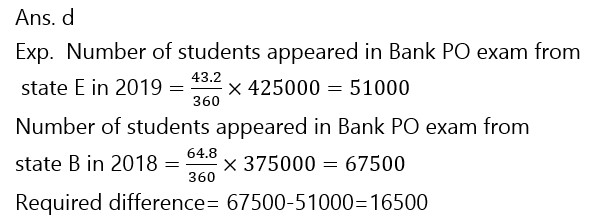1. What is the average number of students appeared in Bank PO exam in 2018 and 2019 together from state D?

(a) 57250

(b) 56725

(c) 57875

(d) 58750

(e) 565001. What is the ratio of total number of students appeared in Bank PO exam from states A, C and D in 2018 to the total number of students appeared from states B, E and F in 2019?

(a) 107:191

(b) 138:187

(c) 129:179

(d) 147:203

(e) 144:197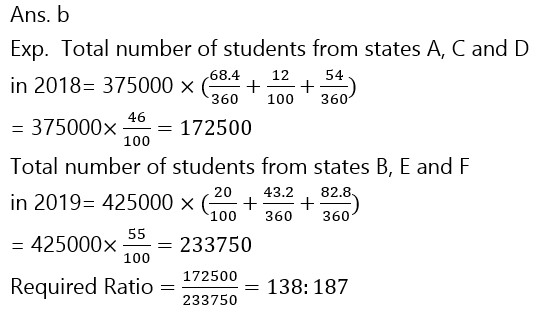1. Number of students appeared in Bank PO exam from state D in 2019 is what percent more/less than the number of students appeared in Bank PO exam from state E in 2018?

(a) 0.83%

(b) 1.12%

(c) 0.69%

(d) 0.76%

(e) 0.91%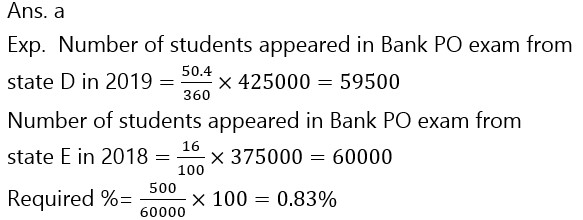Directions (6-10):- In each of the following questions, two equations (I) and (II) are given. Solve the equations and mark the correct option:(a) if x>y

(b) if x≥y

(c) if x<y

(d) if x ≤y

(e) if x = y or no relation can be established between x and y.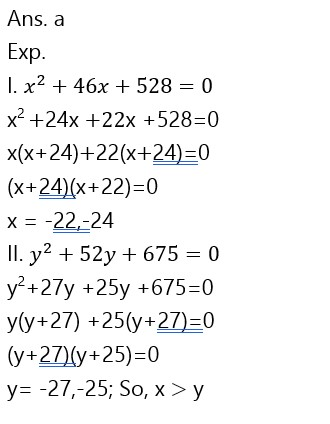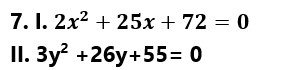(a) if x>y

(b) if x≥y

(c) if x<y

(d) if x ≤y

(e) if x = y or no relation can be established between x and y.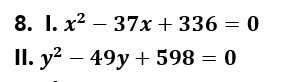(a) if x>y

(b) if x≥y

(c) if x<y

(d) if x ≤y

(e) if x = y or no relation can be established between x and y.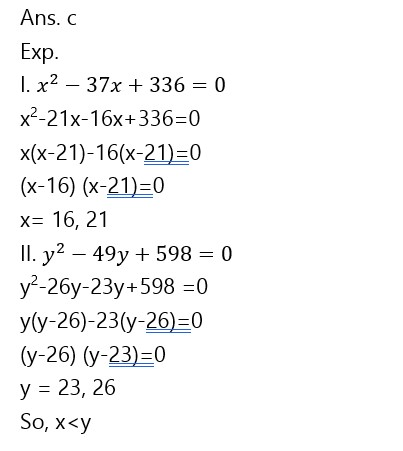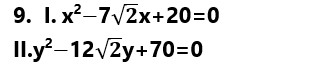(a) if x>y

(b) if x≥y

(c) if x<y

(d) if x ≤y

(e) if x = y or no relation can be established between x and y.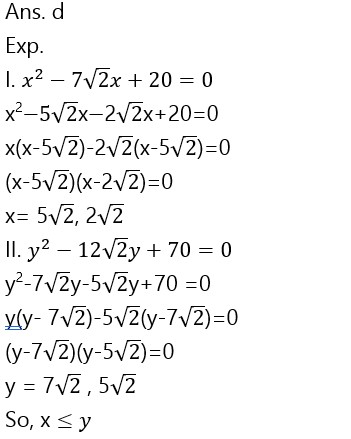(a) if x>y

(b) if x≥y

(c) if x<y

(d) if x ≤y

(e) if x = y or no relation can be established between x and y.